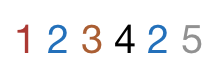# An Even Challenge

Probability Level 2How many numbers of Six digits can be made by arranging the digits of the number $123425$ so that all the even digits do not occupy consecutive places.

Details and Assumptions

• The number is indeed $123425$, there is no typo.
×